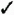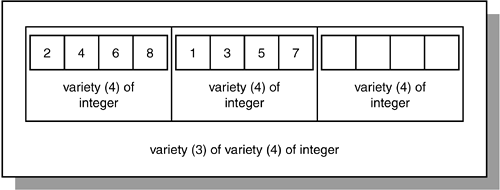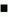# Lab 18.3 Multilevel Collections

## Lab Objective

After this Lab, you will be able to:Use Multilevel Collections

So far you have seen various examples of collections with the element type based on a scalar type, such as NUMBER and VARCHAR2. Starting with Oracle 9 i , PL/SQL provides you with the ability to create collections whose element type is based on a collection type. Such collections are called multilevel collections . You will recall that multilevel collections is a relatively new feature that was introduced in Oracle 9 i .

Consider a varrray of varrays shown in Figure 18.3.

##### Figure 18.3. A Varray of VarraysFigure 18.3 shows a varray of varrays or nested varray. A varray of varrays consists of three elements, where each individual element is a varray consisting of four integer numbers . As a result, in order to reference an individual element of a varray of varrays, you use the following notation:

` `
` varray_name(subscript of the outer varray)(subscript of the inner varray) `

For example, the varray(1)(3) in Figure 18.3 equals 6; similarly, varray(2)(1) equals 1.

Consider an example based on Figure 18.3.FOR EXAMPLE

` `
` DECLARE    TYPE varray_type1 IS VARRAY(4) OF INTEGER;    TYPE varray_type2 IS VARRAY(3) OF varray_type1;    varray1 varray_type1 := varray_type1(2, 4, 6, 8);    varray2 varray_type2 := varray_type2(varray1); BEGIN    DBMS_OUTPUT.PUT_LINE ('Varray of integers');    FOR i IN 1..4 LOOP       DBMS_OUTPUT.PUT_LINE ('varray1('i'): 'varray1(i));    END LOOP;    varray2.EXTEND;    varray2(2) := varray_type1(1, 3, 5, 7);    DBMS_OUTPUT.PUT_LINE (chr(10)'Varray of varrays of integers');    FOR i IN 1..2 LOOP       FOR j IN 1..4 LOOP          DBMS_OUTPUT.PUT_LINE             ('varray2('i')('j'): 'varray2(i)(j));       END LOOP;    END LOOP; END; `

In this declaration portion of the example, you define two varray types. The first type, varray_type1 , is based on the INTEGER datatype and can contain up to four elements. The second type, varray_type2 , is based on the varray_type1 and can contain up to six elements. Next, you declare two varrays based on the types just described. The first varray, varray1 , is declared as varray_type1 and initialized so that its four elements are populated with the first four even numbers. The second varray, varray2 , is declared as varray_type2 , so that each individual element is a varray consisting of four integer numbers, and initialized so that it first varray element is populated.

In the executable portion of the example, you display the values of the varray1 on the screen. Next, you extend the upper bound of the varray2 by one, and populate its second element as follows :

` `
`  varray2(2) := varray_type1(1, 3, 5, 7);  `

Notice that you are using a constructor corresponding to the varray_type1 because each element of the varray2 is based on the varray1 collection. In other words, the same result could be achieved via the following two statements:

` `
`  varray1 := varray_type1(1, 3, 5, 7);   varray2 := varray_type2(varray1);  `

Once the second element of the varray2 is populated, you display results on the screen via nested numeric FOR loops .

This example produces the following output:

` `
`  Varray of integers   varray1(1): 2   varray1(2): 4   varray1(3): 6   varray1(4): 8   Varray of varrays of integers   varray2(1)(1): 2   varray2(1)(2): 4   varray2(1)(3): 6   varray2(1)(4): 8   varray2(2)(1): 1   varray2(2)(2): 3   varray2(2)(3): 5   varray2(2)(4): 7   PL/SQL procedure successfully completed.  `Oracle PL[s]SQL by Example
ISBN: 3642256902
EAN: N/A
Year: 2003
Pages: 289

flylib.com © 2008-2017.
If you may any questions please contact us: flylib@qtcs.net